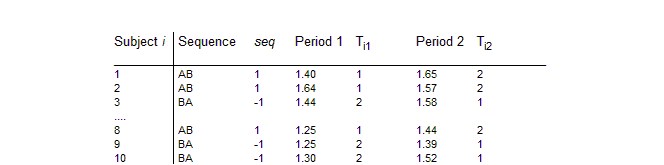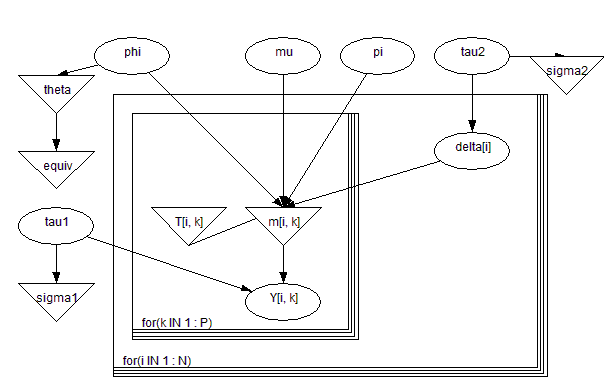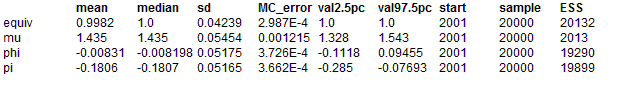#### Equiv: bioequivalence in a cross-over trial

The table below shows some data from a two-treatment, two-period crossover trial to compare 2 tablets A and B, as reported by Gelfand et al (1990).The response Yik from the i th subject (i = 1,...,10) in the k th period (k = 1,2) is assumed to be of the form

Yik ~ Normal(mik, τ1)

mik = μ + (-1)Tik - 1 φ / 2 + (-1)k - 1 π / 2 + δi

δi ~ Normal(0, τ2)

where Tik= 1,2 denotes the treatment given to subject i in period k, μ, φ, π are the overall mean, treatment and period effects respectively, and δi represents the random effect for subject i. The graph of this model and its BUGS language description are shown below

Graphical model for equiv exampleBUGS language for equiv example
model
{
for( k in 1 : P ) {
for( i in 1 : N ) {
Y[i , k] ~ dnorm(m[i , k], tau1)
m[i , k] <- mu + sign[T[i , k]] * phi / 2 + sign[k] * pi / 2 + delta[i]
T[i , k] <- group[i] * (k - 1.5) + 1.5
}
}
for( i in 1 : N ) {
delta[i] ~ dnorm(0.0, tau2)
}
tau1 ~ dgamma(0.001, 0.001) sigma1 <- 1 / sqrt(tau1)
tau2 ~ dgamma(0.001, 0.001) sigma2 <- 1 / sqrt(tau2)
mu ~ dnorm(0.0, 1.0E-6)
phi ~ dnorm(0.0, 1.0E-6)
pi ~ dnorm(0.0, 1.0E-6)
theta <- exp(phi)
equiv <- step(theta - 0.8) - step(theta - 1.2)
}

Note the use of the step function to indicate whether θ = eφ lies between 0.8 and 1.2 which traditionally determines bioequivelence.
##### Data
``` list(N = 10, P = 2,      group = c(1, 1, -1, -1, -1, 1, 1, 1, -1, -1),   Y = structure(.Data = c(1.40, 1.65,                        1.64, 1.57,                           1.44, 1.58,                           1.36, 1.68,                           1.65, 1.69,                           1.08, 1.31,                           1.09, 1.43,                           1.25, 1.44,                           1.25, 1.39,                           1.30, 1.52), .Dim = c(10, 2)),                           sign = c(1, -1)) ```
##### Inits for chain 1
``` list(mu=0, phi=0, pi=0, tau1= 1, tau2 = 1) ```

##### Inits for chain 2
``` list(mu=10, phi=10, pi=10, tau1= 0.1, tau2 = 0.1) ```
Results